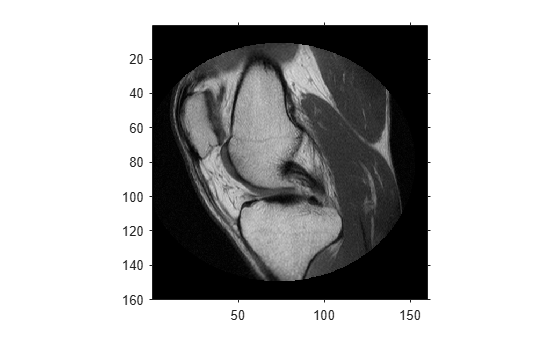# worldToIntrinsic

Convert from world to intrinsic coordinates

## Syntax

``[xIntrinsic, yIntrinsic] = worldToIntrinsic(R,xWorld,yWorld)``
``[xIntrinsic,yIntrinsic,zIntrinsic] = worldToIntrinsic(R,xWorld,yWorld,zWorld)``

## Description

example

````[xIntrinsic, yIntrinsic] = worldToIntrinsic(R,xWorld,yWorld)` maps points from the 2-D world system (`xWorld`,`yWorld`) to the 2-D intrinsic system (`xIntrinsic`,`yIntrinsic`) based on the relationship defined by 2-D spatial referencing object `R`.If the kth input coordinates (`xWorld`(k),`yWorld`(k)) fall outside the image bounds in the world coordinate system, `worldToIntrinsic` extrapolates `xIntrinsic`(k) and `yIntrinsic`(k) outside the image bounds in the intrinsic coordinate system.```

example

````[xIntrinsic,yIntrinsic,zIntrinsic] = worldToIntrinsic(R,xWorld,yWorld,zWorld)` maps points from the world coordinate system to the intrinsic coordinate system using 3-D spatial referencing object `R`.```

## Examples

collapse all

Read a 2-D grayscale image of a knee into the workspace.

```m = dicominfo('knee1.dcm'); A = dicomread(m);```

Create an `imref2d` object, specifying the size and the resolution of the pixels. The DICOM file contains a metadata field `PixelSpacing` that specifies the image resolution in each dimension in millimeters per pixel.

`RA = imref2d(size(A),m.PixelSpacing(2),m.PixelSpacing(1))`
```RA = imref2d with properties: XWorldLimits: [0.1562 160.1562] YWorldLimits: [0.1562 160.1562] ImageSize: [512 512] PixelExtentInWorldX: 0.3125 PixelExtentInWorldY: 0.3125 ImageExtentInWorldX: 160 ImageExtentInWorldY: 160 XIntrinsicLimits: [0.5000 512.5000] YIntrinsicLimits: [0.5000 512.5000] ```

Display the image, including the spatial referencing object. The axes coordinates reflect the world coordinates. Notice that the coordinate (0,0) is in the upper left corner.

```figure imshow(A,RA,'DisplayRange',[0 512])```Select sample points, and store their world x- and y- coordinates in vectors. For example, the first point has world coordinates (38.44,68.75), the second point is 1 mm to the right of it, and the third point is 7 mm below it. The last point is outside the image boundary.

```xW = [38.44 39.44 38.44 -0.2]; yW = [68.75 68.75 75.75 -1];```

Convert the world coordinates to intrinsic coordinates using `worldToIntrinsic`.

`[xI, yI] = worldToIntrinsic(RA,xW,yW)`
```xI = 1×4 123.0080 126.2080 123.0080 -0.6400 ```
```yI = 1×4 220.0000 220.0000 242.4000 -3.2000 ```

The resulting vectors are the intrinsic x- and y- coordinates in units of pixels. Note that the intrinsic coordinate system is continuous, and some returned intrinsic coordinates have noninteger values. Also, `worldToIntrinsic` extrapolates the intrinsic coordinates of the point outside the image boundary.

Read a 3-D volume into the workspace. This image consists of 27 frames of 128-by-128 pixel images.

```load mri; D = squeeze(D); D = ind2gray(D,map);```

Create an `imref3d` spatial referencing object associated with the volume. For illustrative purposes, provide a pixel resolution in each dimension. The resolution is in millimeters per pixel.

`R = imref3d(size(D),2,2,4)`
```R = imref3d with properties: XWorldLimits: [1 257] YWorldLimits: [1 257] ZWorldLimits: [2 110] ImageSize: [128 128 27] PixelExtentInWorldX: 2 PixelExtentInWorldY: 2 PixelExtentInWorldZ: 4 ImageExtentInWorldX: 256 ImageExtentInWorldY: 256 ImageExtentInWorldZ: 108 XIntrinsicLimits: [0.5000 128.5000] YIntrinsicLimits: [0.5000 128.5000] ZIntrinsicLimits: [0.5000 27.5000] ```

Select sample points, and store their world x-, y-, and z-coordinates in vectors. For example, the first point has world coordinates (108,92,52), the second point is 3 mm above it in the +z-direction, and the third point is 0.2 mm to the right of it in the +x-direction. The last point is outside the image boundary.

```xW = [108 108 108.2 2]; yW = [92 92 92 -1]; zW = [52 55 52 0.33];```

Convert the world coordinates to intrinsic coordinates using `worldToIntrinsic`.

`[xI, yI, zI] = worldToIntrinsic(R,xW,yW,zW)`
```xI = 1×4 54.0000 54.0000 54.1000 1.0000 ```
```yI = 1×4 46.0000 46.0000 46.0000 -0.5000 ```
```zI = 1×4 13.0000 13.7500 13.0000 0.0825 ```

The resulting vectors are the intrinsic x-, y-, and z-coordinates in units of pixels. Note that the intrinsic coordinate system is continuous, and some returned intrinsic coordinates have noninteger values. Also, `worldToIntrinsic` extrapolates the intrinsic coordinates of the point outside the image boundary.

## Input Arguments

collapse all

Spatial referencing object, specified as an `imref2d` or `imref3d` object.

Coordinates along the x-dimension in the world coordinate system, returned as a numeric scalar or vector.

Data Types: `single` | `double` | `int8` | `int16` | `int32` | `int64` | `uint8` | `uint16` | `uint32` | `uint64`

Coordinates along the y-dimension in the world coordinate system, returned as a numeric scalar or vector. `yWorld` is the same length as `xWorld`.

Data Types: `single` | `double` | `int8` | `int16` | `int32` | `int64` | `uint8` | `uint16` | `uint32` | `uint64`

Coordinates along the z-dimension in the world coordinate system, returned as a numeric scalar or vector. `zWorld` is the same length as `xWorld`.

Data Types: `single` | `double` | `int8` | `int16` | `int32` | `int64` | `uint8` | `uint16` | `uint32` | `uint64`

## Output Arguments

collapse all

Coordinates along the x-dimension in the intrinsic coordinate system, specified as a numeric scalar or vector. `xIntrinsic` is the same length as `xWorld`.

Data Types: `single` | `double` | `int8` | `int16` | `int32` | `int64` | `uint8` | `uint16` | `uint32` | `uint64`

Coordinates along the y-dimension in the intrinsic coordinate system, specified as a numeric scalar or vector. `yIntrinsic` is the same length as `xWorld`.

Data Types: `single` | `double` | `int8` | `int16` | `int32` | `int64` | `uint8` | `uint16` | `uint32` | `uint64`

Coordinates along the z-dimension in the intrinsic coordinate system, specified as a numeric scalar or vector. `zIntrinsic` is the same length as `xWorld` and `yWorld`.

Data Types: `single` | `double` | `int8` | `int16` | `int32` | `int64` | `uint8` | `uint16` | `uint32` | `uint64`

## Version History

Introduced in R2013a